##帐号 自动登录 找回密码 密码 立即注册→加入我们
 搜索 热搜: 下载 VB C 实现 编写

# 【FPGA】FPGA开发学习之路——自制显卡

1062主题 6万积分

UID
1

221

358 点

19614 个

40564 次

0 份

1855 小时

2014-1-26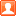发表于 2019-12-15 00:42:43 | 显示全部楼层 |阅读模式

### 欢迎访问技术宅的结界，请注册或者登录吧。

x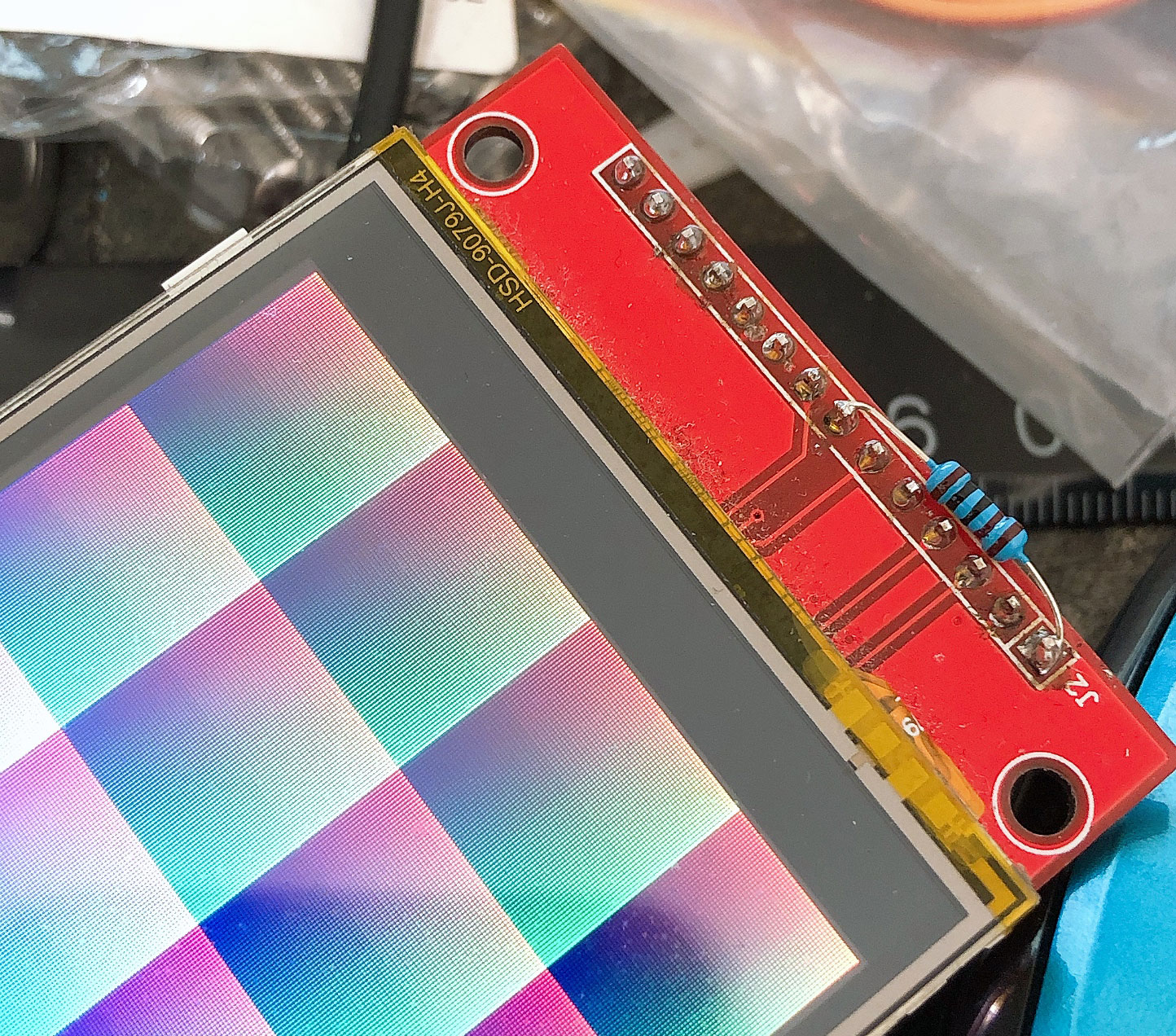1、接受绘制命令，根据绘制参数进行绘制，并且有自己的指令集用于编码着色器，然后应用流水线运行着色器指令进行并行计算。
2、具备一定的存储空间，可存储材质纹理，图元、图形，以及着色器指令。
3、使用一部分存储空间用于存储帧缓存。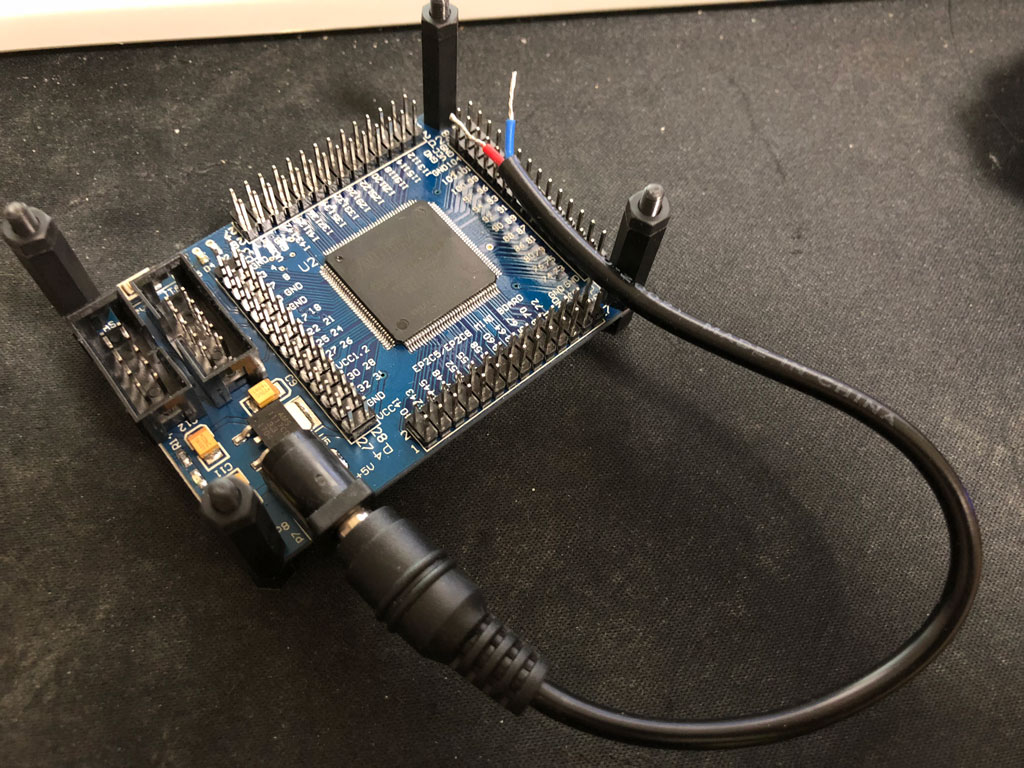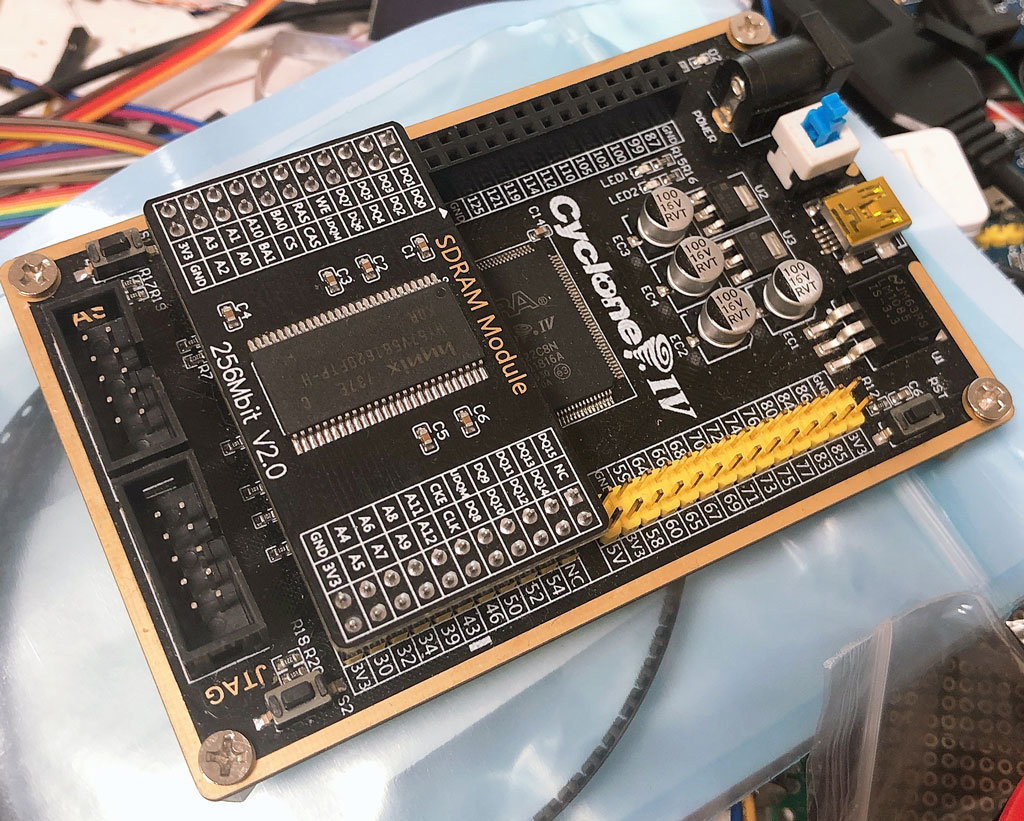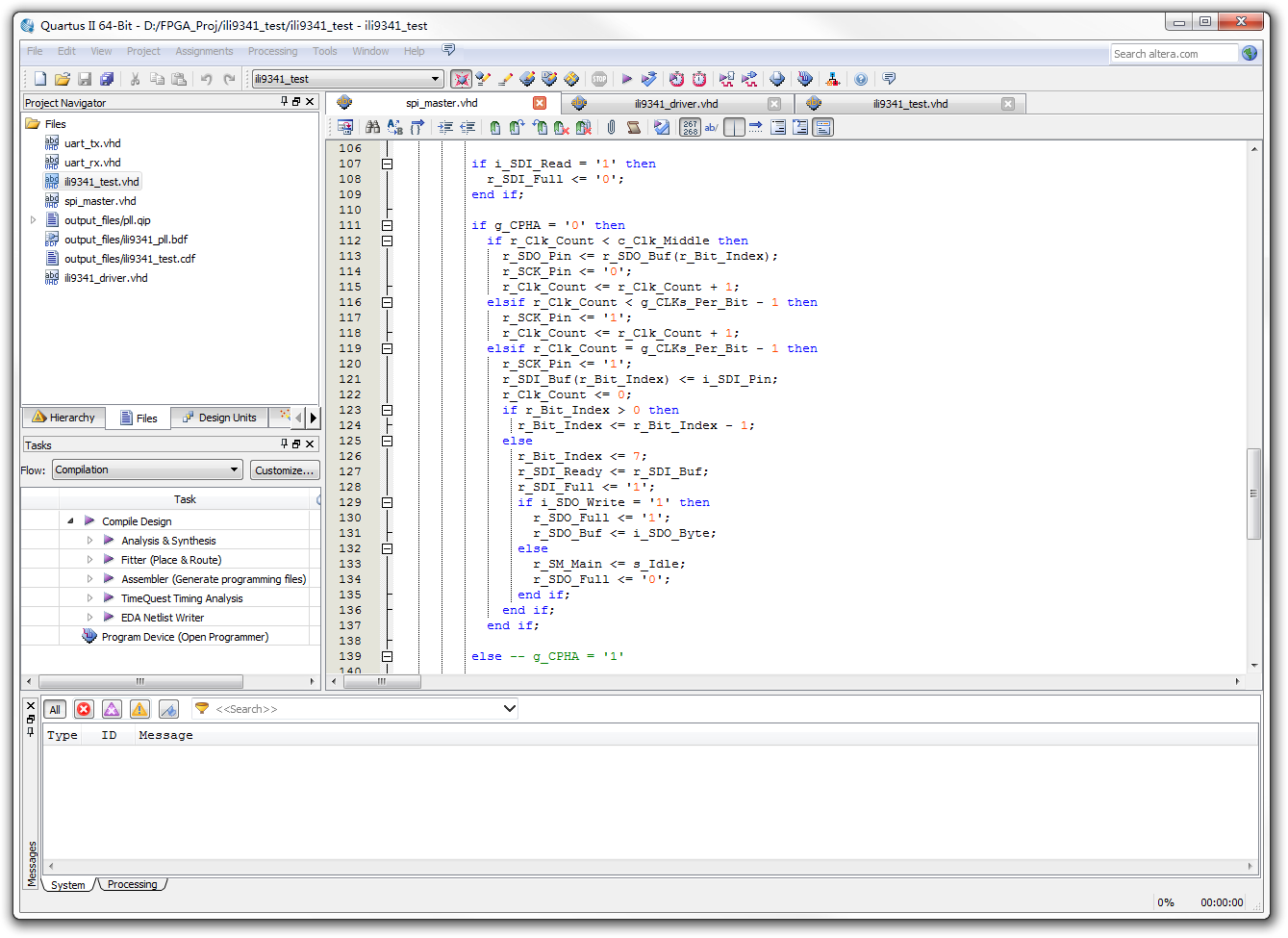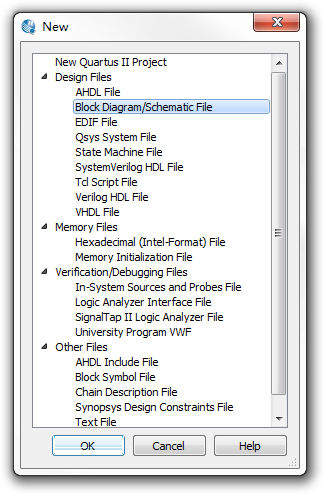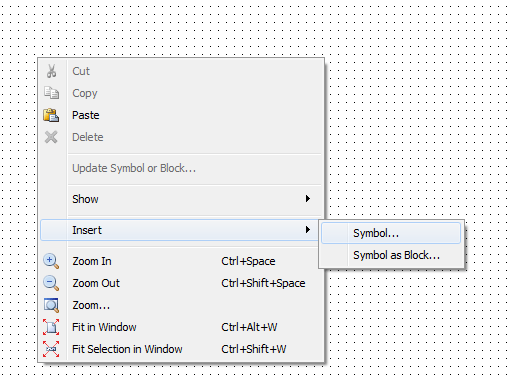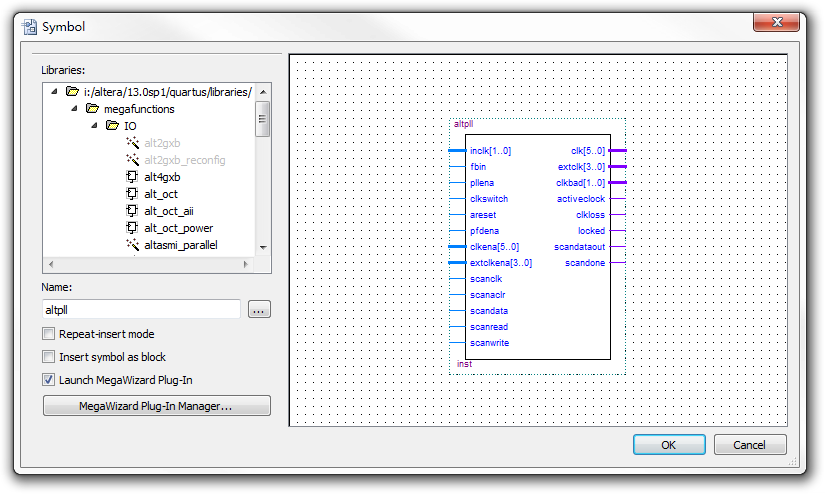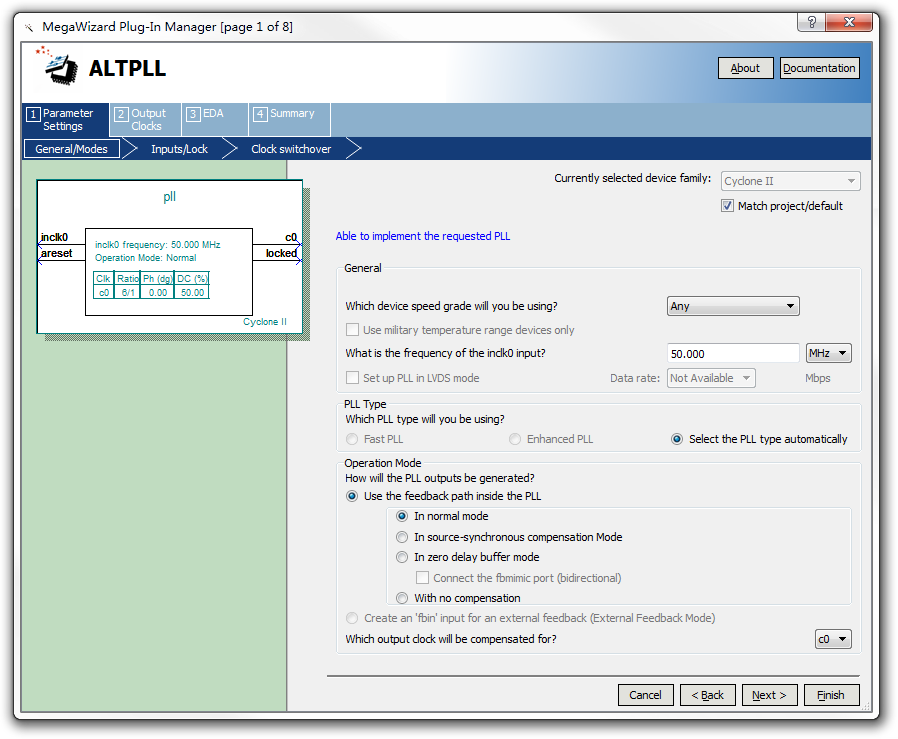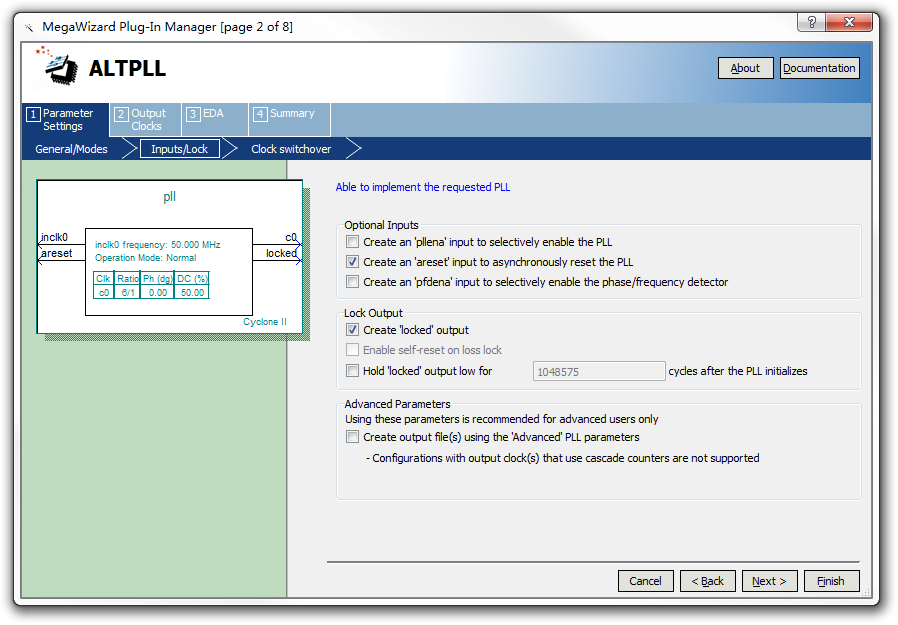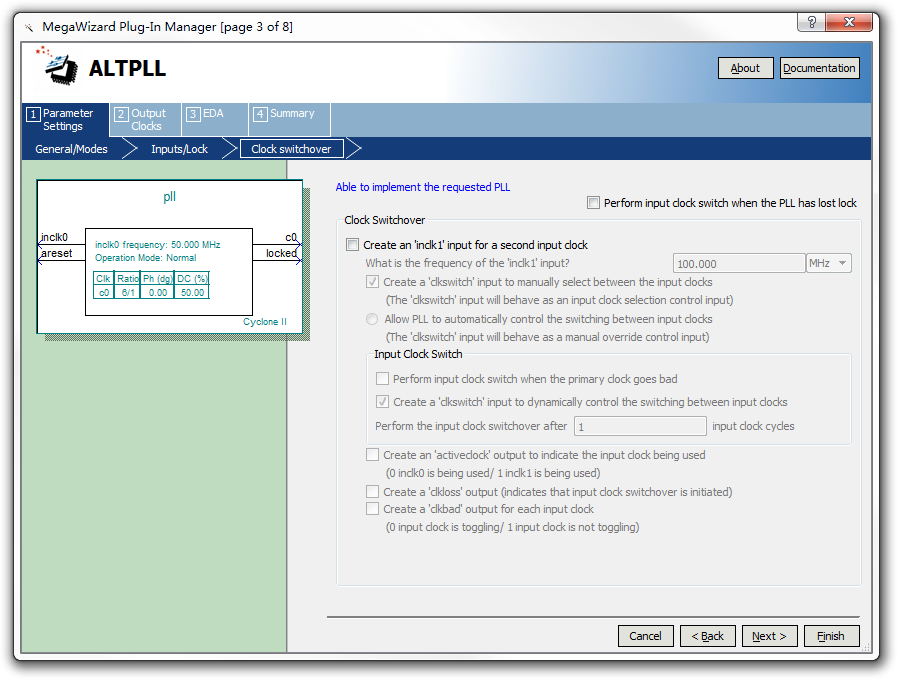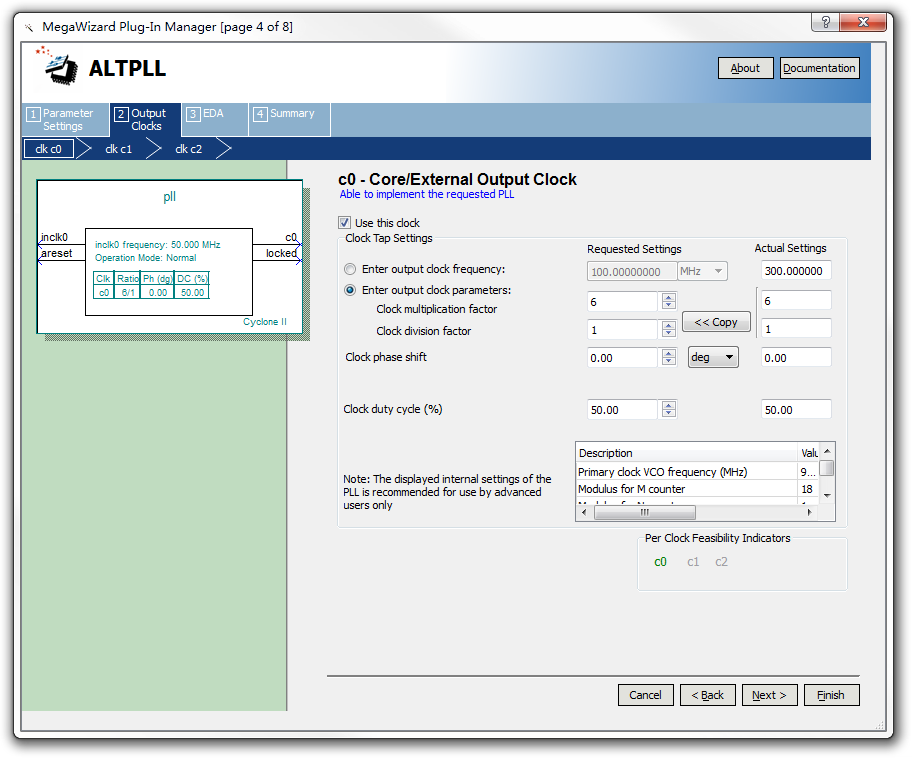ALTPLL能输出不止一个的时钟信号，不过暂时我们似乎不需要（反正需要的时候，自己在自己的components里把它写上就行了）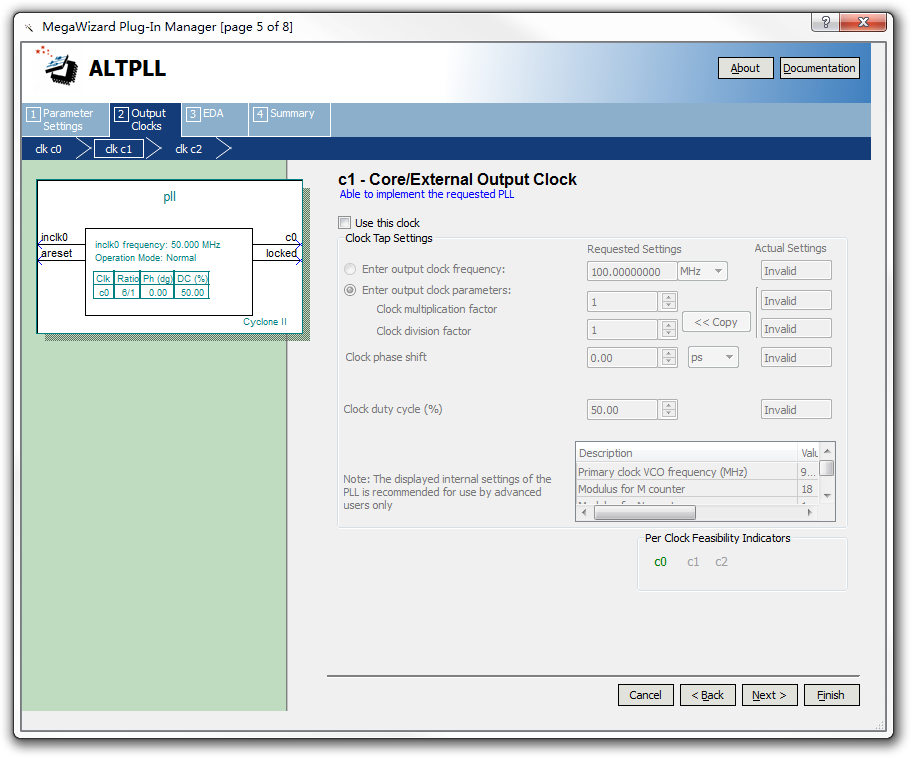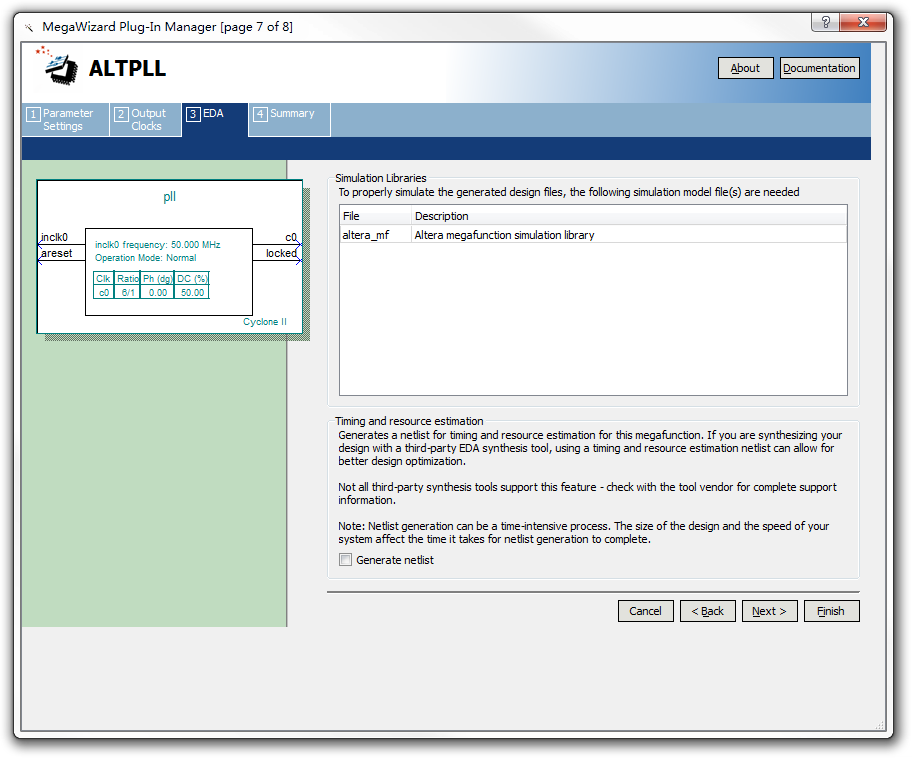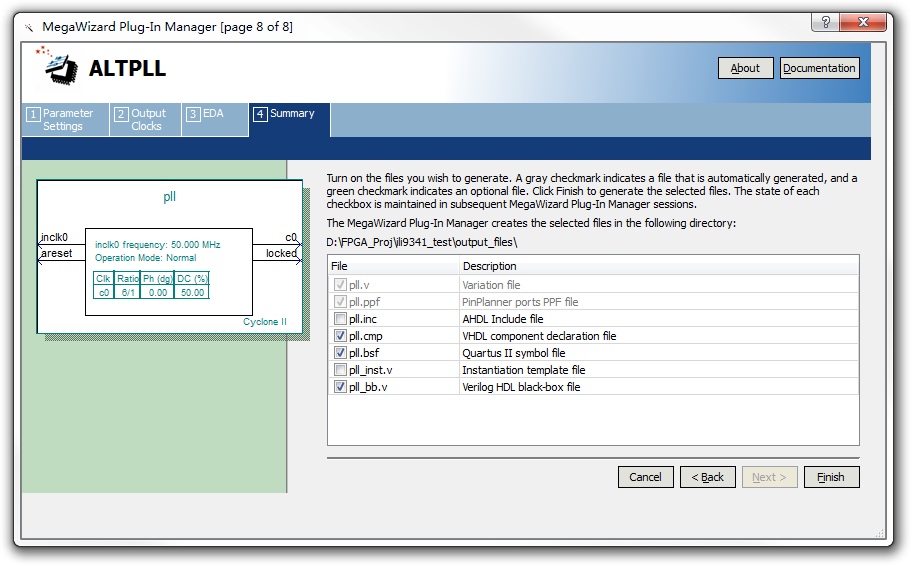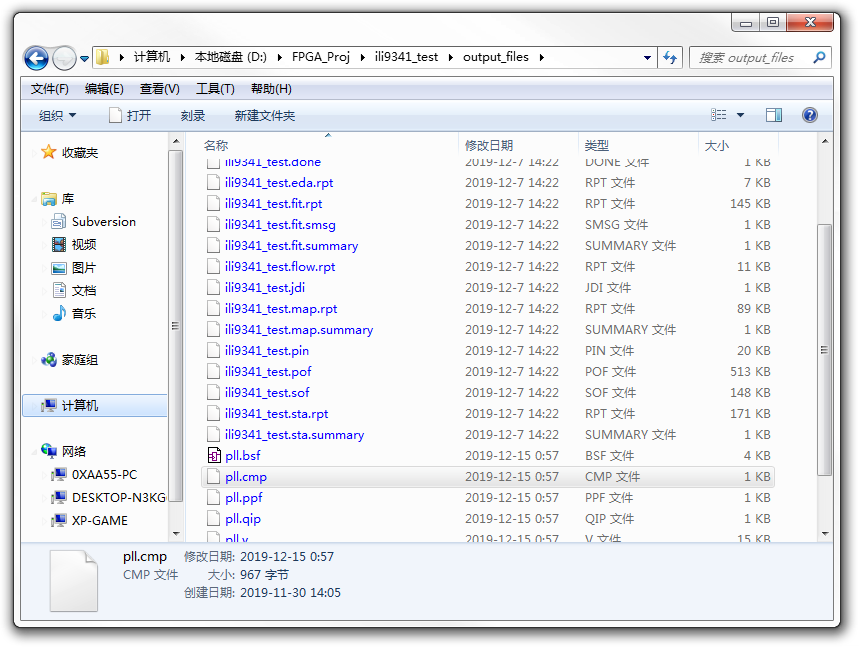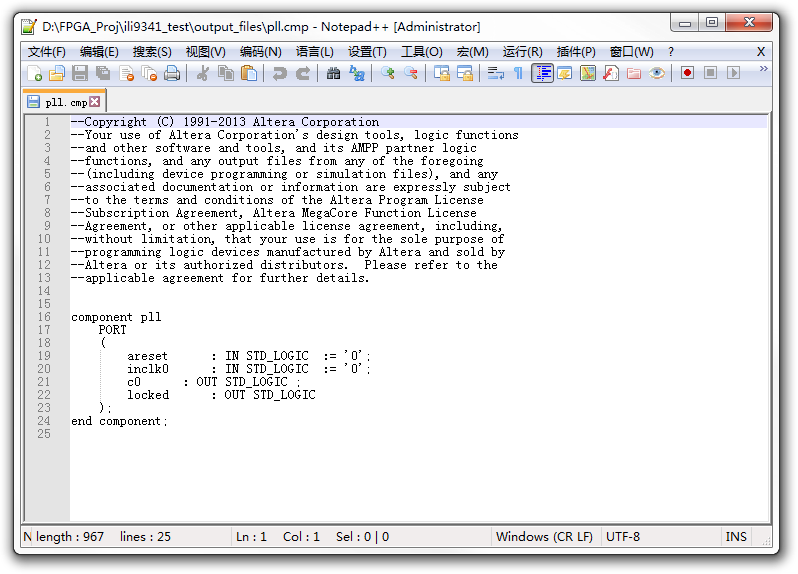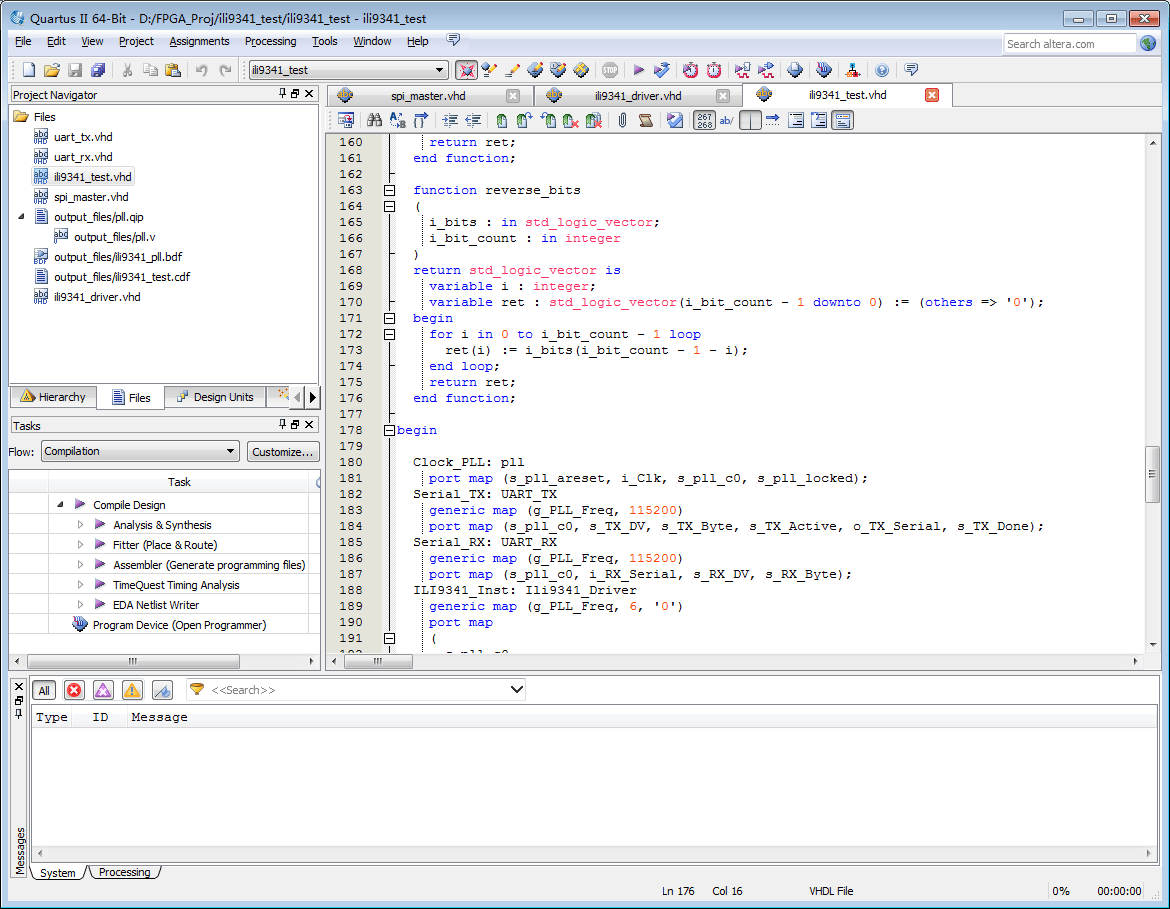library ieee;
use ieee.std_logic_1164.ALL;
use IEEE.std_logic_unsigned.all;
use ieee.numeric_std.all;

entity spi_master is
generic
(
g_CLKs_Per_Bit  : INTEGER := 2;
g_CPOL : std_logic := '0';
g_CPHA : std_logic := '0'
);
port
(
i_Clk        : in  std_logic;
i_Reset      : in  std_logic;
o_Active     : out std_logic;
i_SDO_Write  : in  std_logic;
i_SDO_Byte   : in  std_logic_vector(7 downto 0);
o_SDO_Full   : out std_logic;
o_SDI_Byte   : out std_logic_vector(7 downto 0);
o_SDI_Full   : out std_logic;
o_Bit_Index  : out integer;

o_NSS_Pin : out std_logic;
o_SCK_Pin : out std_logic;
o_SDO_Pin : out std_logic;
i_SDI_Pin : in  std_logic
);
end spi_master;

architecture rtl of spi_master is

type t_SM_Main is (s_Idle, s_Active);
signal r_SM_Main : t_SM_Main := s_Idle;

signal r_Clk_Count    : integer range 0 to g_CLKs_Per_Bit - 1:= 0;
constant c_Clk_Middle : integer := g_CLKs_Per_Bit / 2;

signal r_Bit_Index    : integer range 0 to 7;  -- 8 Bits Total
signal r_SDO_Buf      : std_logic_vector(7 downto 0) := (others => '0');
signal r_SDI_Buf      : std_logic_vector(7 downto 0) := (others => '0');
signal r_SDI_Ready    : std_logic_vector(7 downto 0) := (others => '0');
signal r_SDO_Full     : std_logic := '0';
signal r_SDI_Full     : std_logic := '0';

signal r_NSS_Pin   : std_logic := '0';
signal r_SDO_Pin   : std_logic := '0';
signal r_SCK_Pin   : std_logic := '0';

begin

o_SDO_Full <= r_SDO_Full;
o_SDI_Full <= r_SDI_Full;
o_Bit_Index <= r_bit_Index;

o_SDO_Pin <= r_SDO_Pin;
o_SCK_Pin <= r_SCK_Pin xor g_CPOL;
o_NSS_Pin <= r_NSS_Pin;

p_SPI_XCV: process (i_Clk)
begin
if rising_edge(i_Clk) then
if i_Reset = '1' then
r_SM_Main <= s_Idle;
r_Clk_Count <= 0;
r_Bit_Index <= 7;
r_SDI_Full <= '0';
r_SDO_Full <= '0';
o_Active <= '0';
r_NSS_Pin <= '1';
r_SCK_Pin <= '0';

else case r_SM_Main is

when s_Idle =>
r_Clk_Count <= 0;
r_Bit_Index <= 7;
r_SCK_Pin <= '0';
r_SDO_Full <= '0';

r_SDI_Full <= '0';
end if;

if i_SDO_Write = '1' then
r_SDO_Full <= '1';
r_NSS_Pin <= '0';
o_Active <= '1';
r_SDO_Buf <= i_SDO_Byte;
r_SM_Main <= s_Active;
if g_CPHA = '0' then
r_SDO_Pin <= i_SDO_Byte(r_Bit_Index);
end if;
else
r_NSS_Pin <= '1';
o_Active <= '0';
r_SM_Main <= s_Idle;
end if;

when s_Active =>
r_NSS_Pin <= '0';

r_SDI_Full <= '0';
end if;

if g_CPHA = '0' then
if r_Clk_Count < c_Clk_Middle then
r_SDO_Pin <= r_SDO_Buf(r_Bit_Index);
r_SCK_Pin <= '0';
r_Clk_Count <= r_Clk_Count + 1;
elsif r_Clk_Count < g_CLKs_Per_Bit - 1 then
r_SCK_Pin <= '1';
r_Clk_Count <= r_Clk_Count + 1;
elsif r_Clk_Count = g_CLKs_Per_Bit - 1 then
r_SCK_Pin <= '1';
r_SDI_Buf(r_Bit_Index) <= i_SDI_Pin;
r_Clk_Count <= 0;
if r_Bit_Index > 0 then
r_Bit_Index <= r_Bit_Index - 1;
else
r_Bit_Index <= 7;
r_SDI_Full <= '1';
if i_SDO_Write = '1' then
r_SDO_Full <= '1';
r_SDO_Buf <= i_SDO_Byte;
else
r_SM_Main <= s_Idle;
r_SDO_Full <= '0';
end if;
end if;
end if;

else -- g_CPHA = '1'

if r_Clk_Count < c_Clk_Middle then
r_SCK_Pin <= '0';
r_Clk_Count <= r_Clk_Count + 1;
elsif r_Clk_Count < g_CLKs_Per_Bit - 1 then
r_SCK_Pin <= '1';
r_SDO_Pin <= r_SDO_Buf(r_Bit_Index);
r_Clk_Count <= r_Clk_Count + 1;
elsif r_Clk_Count = g_CLKs_Per_Bit - 1 then
r_SCK_Pin <= '1';
r_SDO_Pin <= r_SDO_Buf(r_Bit_Index);
r_SDI_Buf(r_Bit_Index) <= i_SDI_Pin;
r_Clk_Count <= 0;
if r_Bit_Index > 0 then
r_Bit_Index <= r_Bit_Index - 1;
else
r_Bit_Index <= 7;
r_SDI_Full <= '1';
if i_SDO_Write = '1' then
r_SDO_Full <= '1';
r_SDO_Buf <= i_SDO_Byte;
else
r_SM_Main <= s_Idle;
r_SDO_Full <= '0';
end if;
end if;
end if;

end if; -- g_CPHA

when others =>
r_SM_Main <= s_Idle;

end case; end if;
end if;
end process p_SPI_XCV;

end rtl;
（知道为什么这个代码的缩进那么丑吗？因为在Quartus II里面，TAB是3个字符长度的，而在这里它是8个字符）

### 相关帖子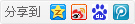1主题 285积分

UID
2054

0

17 点

197 个

20 次

0 份

24 小时

2016-11-10发表于 2019-12-15 02:38:38 | 显示全部楼层
 本帖最后由 大能猫 于 2019-12-15 03:12 编辑 硬件日常玄学问题（捂脸 然后，emmm。。。不是IO口速率不够而是IO口驱动能力不够。因为IO口以高频率输出时（比如这里的CLK）相当于一个高频交流电，所以容抗往往会比较大，需要更大的电流才能正确地输出电平（不知道这样讲准不准确 这也是为什么频率越高的东西一般电压越低而且功耗会高 这里上拉电阻主要起到的作用就是补偿电流，因为当IO口电平由低变高时，IO口推挽输出的两个晶体管上管导通（上管与VDD连），下管关闭（下管与GND连），IO口驱动电流不够的原因就是上管导通时电流不够（很大原因是IO口一般是通过一根限流电阻与VDD连接的，这样能保证IO口直接接地也不会烧芯片），导致无法在IO口由高变低前让IO口的电压达到逻辑1的阈值电压（上面说了高频下容抗大，导线和晶体管都是有电容的），这时候如果在导线上接一根到VDD的电阻，就相当于跟IO口内部的电阻并联了，就增加了输入电流。 然后还有一个问题就是上拉电阻不会影响低电平么，因为照理说在IO口由高变低时是导线电容放电过程，而上拉电阻在这过程中间也会补偿放电电流，如果推挽输出的下管跟上管一样接了一根电阻不会使电平跳变前无法下降到逻辑0的阈值电压么 答案是一般不会，因为上管接电阻是防止驱动电流过大烧芯片，而下管一般来说没必要再做限流（感觉有点类似于保险丝接火线不接零线的想法），可能也跟制片的时候地的面积一般会比VDD大很多所以能过的电流 也大（这个原因是猜的），还有个可能的原因是如果下端晶体管电阻过大，而与其连接的IO口阻抗又太小会影响低电平的输出（导致IO口上的分压高于逻辑0的阈值电压）。总之下管一般电阻是比较小的，所以不存在IO口放电能力不够的情况 PS：最后，一般所有的空格和编码问题我都建议用vscode（逃

### 评分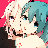0xAA55 + 5 + 20 + 10 回答很详细

1062主题 6万积分

UID
1

221

358 点

19614 个

40564 次

0 份

1855 小时

2014-1-26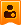楼主| 发表于 2019-12-15 08:15:51 | 显示全部楼层
 我其实也思考过如果接了个上拉电阻，它是不是就拉不低了。或者，我这里接了的上拉电阻，是不是起到的作用是让波形更“居中”从而被辨识。但，根据大能猫老兄的回答，应该是让眼图更睁开了的样子。或者说，可能因为下拉电阻阻值小，这个上拉电阻并没有让它线性增加输出电平吧。不过我觉得下拉电阻小的原因应该是这个芯片想让自己的脚支持开漏方式的多设备共享某一总线的功能。 忽然想到，“眼图”这个词用日语怎么说呢？“眼睛”我知道是「目」（め、me），“图”则是「図」（ず、zu），连起来是「目図」，读音听起来像“妹子”……

1主题 285积分

UID
2054

0

17 点

197 个

20 次

0 份

24 小时

2016-11-10发表于 2019-12-15 21:22:06 | 显示全部楼层
 0xAA55 发表于 2019-12-15 08:15 我其实也思考过如果接了个上拉电阻，它是不是就拉不低了。或者，我这里接了的上拉电阻，是不是起到的作用是 ... 应该是这样 开漏的话也是原因，如果总线上的阻抗不够就会导致电平拉不下来，也是类似我上面说的第三个理由（不过现在的IO口似乎OD模式和推挽输出模式是会接到不同电路上的）

1062主题 6万积分

UID
1

221

358 点

19614 个

40564 次

0 份

1855 小时

2014-1-26楼主| 发表于 2019-12-15 21:26:57 | 显示全部楼层
 大能猫 发表于 2019-12-15 21:22 应该是这样 开漏的话也是原因，如果总线上的阻抗不够就会导致电平拉不下来，也是类似我上面说的第三个理 ... 我的FPGA板子的Pin似乎不能配置开漏还是推拉的模式

1主题 285积分

UID
2054

0

17 点

197 个

20 次

0 份

24 小时

2016-11-10发表于 2019-12-16 03:15:47 | 显示全部楼层
 0xAA55 发表于 2019-12-15 21:26 我的FPGA板子的Pin似乎不能配置开漏还是推拉的模式 OD一个比较主要的用途是转换电平标准，比如一个IO口输出5V为高，要接到3.3V为高的IO口上电压太大了，就可以用OD输出，然后上拉一根电阻到3.3V电源。但fpga本身IO就支持大多数的电平标准，这可能是不支持OD模式的主要原因吧

1062主题 6万积分

UID
1

221

358 点

19614 个

40564 次

0 份

1855 小时

2014-1-26楼主| 发表于 2019-12-16 04:39:48 | 显示全部楼层
 大能猫 发表于 2019-12-16 03:15 OD一个比较主要的用途是转换电平标准，比如一个IO口输出5V为高，要接到3.3V为高的IO口上电压太大了，就可 ... 原来如此，我其实虽然知道它们的差异，但我并不知道OD兼容不同电平的情况。 大能猫大佬果然见多识广

1主题 285积分

UID
2054

0

17 点

197 个

20 次

0 份

24 小时

2016-11-10发表于 2019-12-19 04:37:38 | 显示全部楼层
 0xAA55 发表于 2019-12-16 04:39 原来如此，我其实虽然知道它们的差异，但我并不知道OD兼容不同电平的情况。 大能猫大佬果然见多识广 ... 过。。。过奖了，就是一些所谓的常识，不知道只是因为没听说过一旦听说过很自然就可以理解为什么，不值一夸（被A5大佬夸有点不知所措）

 本版积分规则 回帖并转播 回帖后跳转到最后一页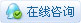|申请友链||Archiver|手机版|小黑屋|技术宅的结界 ( 滇ICP备16008837号 )|网站地图

GMT+8, 2020-8-9 05:29 , Processed in 0.112539 second(s), 36 queries , Gzip On.